• 机器学习概念简介
• 常用机器学习算法和过程
• 特征提取
• 支持向量机(SVM)
• 训练和预测

# 机器学习概念简介

The machine learning definition by Arthur Samuel is referenced in Some Studies in Machine Learning Using the Game of Checkers in IBM Journal of Research and Development (Volume3, Issue: 3), p. 210. It was also referenced in The New Yorker and Office Management in the same year.  The more formal definition from Tom M. Mitchel is referenced in Machine Learning Book, McGray Hill 1997: (http://www.cs.cmu.edu/afs/cs.cmu.edu/user/mitchell/ftp/mlbook.html).

• 监督学习：计算机从一组标记的数据中学习。 这里的目标是了解允许计算机映射数据和输出标签结果之间关系的模型和规则的参数。

• 无监督学习：不给出标签，计算机试图发现给定数据的输入结构。

• 强化学习：计算机与动态环境交互，达到他们的目标并从他们的错误中学习。

• 分类：输入的空间可以分为四个个类，给定样本的预测结果就是这些训练类中的一个。 这是最常用的类别之一。 一个典型的例子可能是电子邮件垃圾邮件过滤，其中只有两类：垃圾邮件和非垃圾邮件。 或者，我们可以使用 OCR，其中只有 N 个字符可用，并且每个字符是一个类。
• 回归：输出是连续值，而不是像分类结果那样的离散值。 回归的一个例子可能是根据房子的大小、建造年限和位置对房价进行预测。
• 聚类：输入将被分成 N 个组，这通常是使用无监督训练来完成的。
• 密度估计：找出输入的(概率)分布。

# OpenCV 机器学习算法

OpenCV 实现了其中八种机器学习算法。 它们都继承自StatModel类：

• 人工神经网络
• 随机树
• 期望最大化
• K-近邻
• Logistic 回归
• 正态贝叶斯分类器

• 支持向量机

• 随机梯度下降支持向量机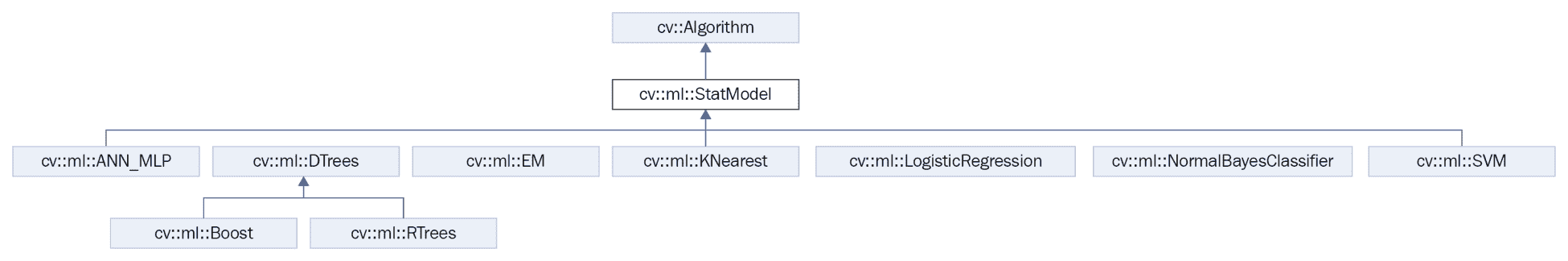StatModel类是所有机器学习算法的基类。 这提供了预测和所有读写功能，这些功能对于保存和读取我们的机器学习参数和训练数据非常重要。

StatModel是除深度学习方法以外的所有机器学习类(如 SVM 或 ANN)的基类。StatModel基本上是一个虚拟类，它定义了两个最重要的函数-trainpredicttrain方法是负责使用训练数据集学习模型参数的主要方法。 此版本有以下三种可能的调用：

bool train(const Ptr<TrainData>& trainData, int flags=0 );
bool train(InputArray samples, int layout, InputArray responses);
Ptr<_Tp> train(const Ptr<TrainData>& data, int flags=0 );


• TrainData：可以从TrainData类加载或创建的培训数据。 这个类是 OpenCV 3 中的新增类，帮助开发人员从机器学习算法中创建训练数据和摘要。 这样做是因为不同的算法需要不同类型的阵列结构来进行训练和预测，例如 ANN 算法。
• samples：训练数组样本的数组，例如机器学习算法要求的格式的训练数据。
• layoutROW_SAMPLE(训练样本为矩阵行)或COL_SAMPLE(训练样本为矩阵列)。
• responses：与样本数据关联的响应向量。
• flags：每个方法定义的可选标志。

predict方法要简单得多，并且只有一个可能的调用：

float StatModel::predict(InputArray samples, OutputArray results=noArray(), int flags=0)


• samples：用于预测模型结果的输入样本可以由任意数量的数据组成，无论是单个数据还是多个数据。
• results：每个输入行样本的结果(由来自先前训练的模型的算法计算)。
• flags：这些可选标志取决于型号。 某些模型(如 Boost)由 SVM 的StatModel::RAW_OUTPUT标志识别，该标志使方法返回原始结果(总和)，而不是类标签。

StatModel类为其他非常有用的方法提供了一个接口：

# 计算机视觉与机器学习工作流

1. 预处理
2. 分段
3. 特征提取
4. 分类结果
5. P主进程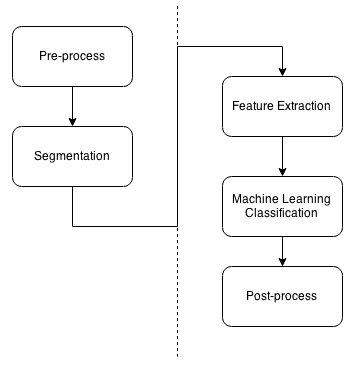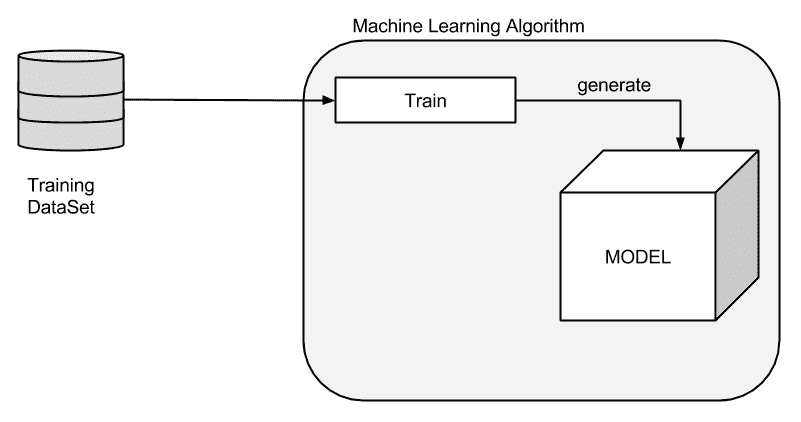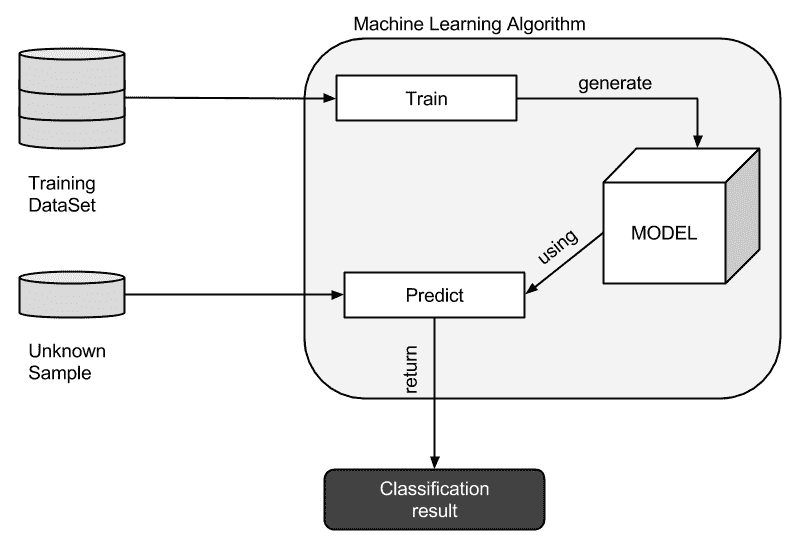# 物体自动检测分类示例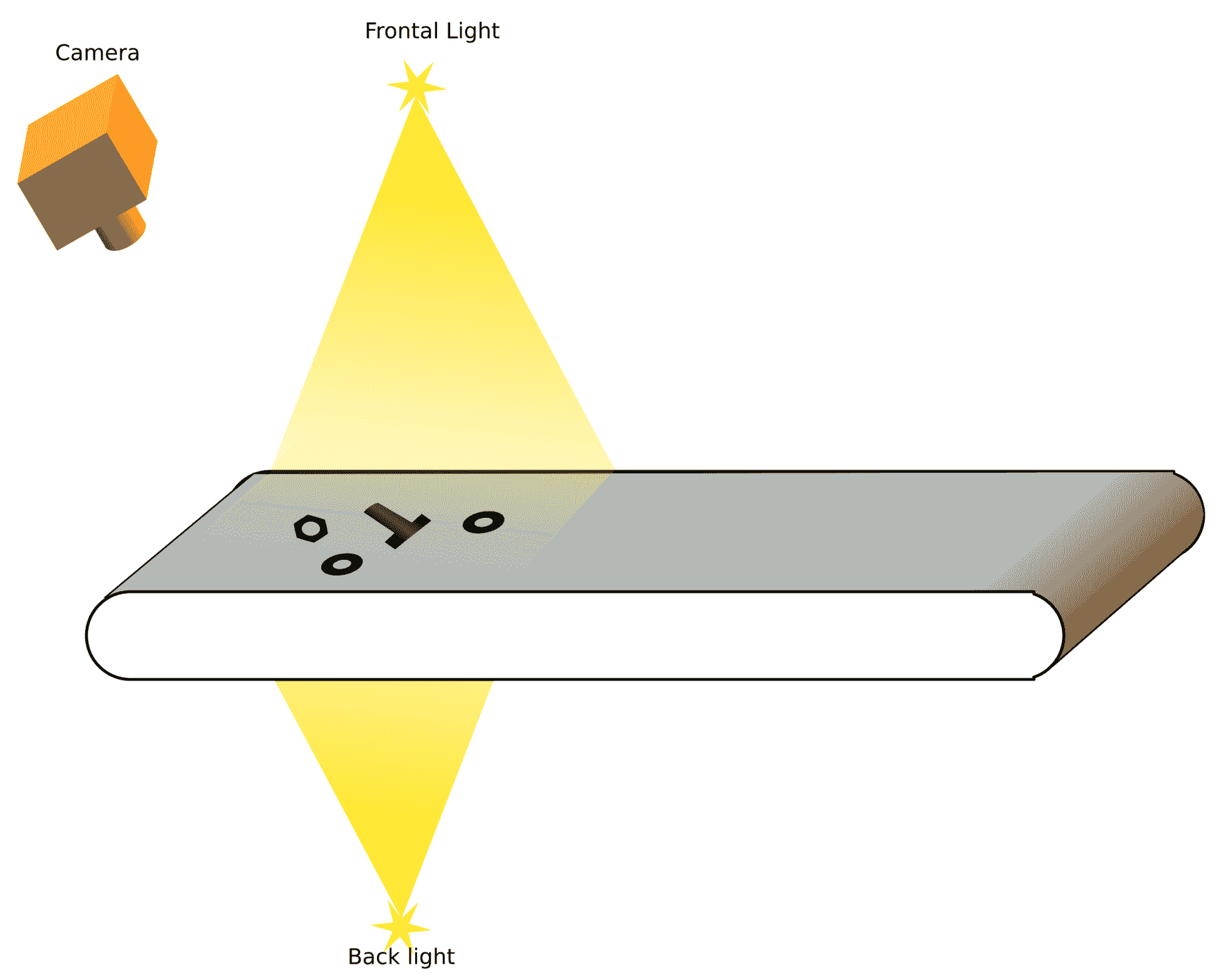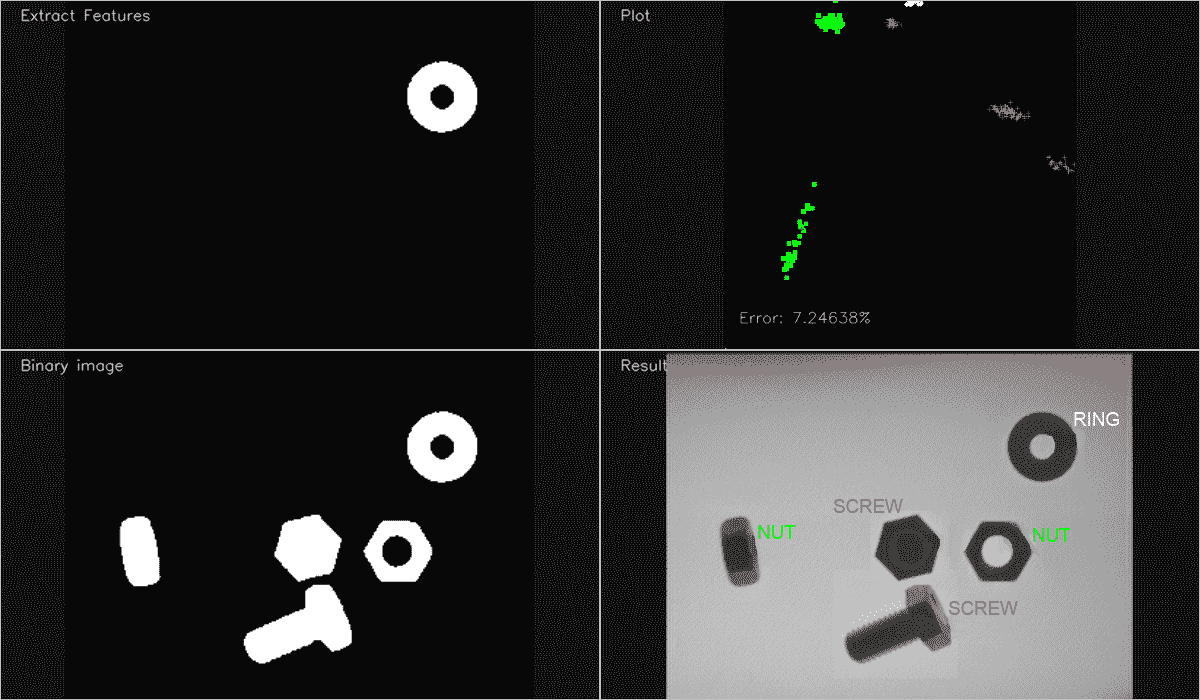1. 对于每个输入图像：

• 对图像进行预处理
• 分割图像
• 对于图像中的每个对象：
• 提取要素
• 将特征添加到带有相应标签(螺母、螺钉、环)的训练特征向量
• 创建一个 SVM 模型。
• 用训练后的特征向量训练支持向量机模型。
• 对输入图像进行预处理，对每个分割对象进行分类。
• 分割输入图像。
• 对于检测到的每个对象：
• 提取要素
• 用支持向量机进行预测
• 模型 / 模式 / 模范 / 时装模特儿
• 在输出图像中绘制结果

# 特征提取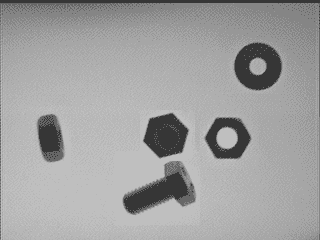• 对象的面积
• 长宽比，即宽度除以边界矩形的高度
• 孔洞的数量
• 等高线边数

vector< vector<float> > ExtractFeatures(Mat img, vector<int>* left=NULL, vector<int>* top=NULL)
{
vector< vector<float> > output;
vector<vector<Point> > contours;
Mat input= img.clone();

vector<Vec4i> hierarchy;
findContours(input, contours, hierarchy, RETR_CCOMP, CHAIN_APPROX_SIMPLE);
// Check the number of objects detected
if(contours.size() == 0){
return output;
}
RNG rng(0xFFFFFFFF);
for(auto i=0; i<contours.size(); i++){

drawContours(mask, contours, i, Scalar(1), FILLED, LINE_8, hierarchy, 1);
float area= area_s;

if(area>500){ //if the area is greater than min.

RotatedRect r= minAreaRect(contours[i]);
float width= r.size.width;
float height= r.size.height;
float ar=(width<height)?height/width:width/height;

vector<float> row;
row.push_back(area);
row.push_back(ar);
output.push_back(row);
if(left!=NULL){
left->push_back((int)r.center.x);
}
if(top!=NULL){
top->push_back((int)r.center.y);
}

// Add image to the multiple image window class, See the class on full github code
miw->render();
waitKey(10);
}
}
return output;
}


  vector< vector<float> > output;
vector<vector<Point> > contours;
Mat input= img.clone();
vector<Vec4i> hierarchy;
findContours(input, contours, hierarchy, RETR_CCOMP, CHAIN_APPROX_SIMPLE);


if(contours.size() == 0){
return output;
}


for(auto i=0; i<contours.size(); i++){
drawContours(mask, contours, i, Scalar(1), FILLED, LINE_8, hierarchy, 1);


    Scalar area_s= sum(mask);
float area= area_s;


if(area>MIN_AREA){ //if the area is greater than min.
RotatedRect r= minAreaRect(contours[i]);
float width= r.size.width;
float height= r.size.height;
float ar=(width<height)?height/width:width/height;


vector<float> row;
row.push_back(area);
row.push_back(ar);
output.push_back(row);


  if(left!=NULL){
left->push_back((int)r.center.x);
}
if(top!=NULL){
top->push_back((int)r.center.y);
}


      miw->addImage("Extract Features", mask*255);
miw->render();
waitKey(10);
}
}
return output;


# 训练支持向量机模型

Ptr<SVM> svm;


void trainAndTest()
{
vector< float > trainingData;
vector< int > responsesData;
vector< float > testData;
vector< float > testResponsesData;

int num_for_test= 20;

// Get the nut images
readFolderAndExtractFeatures("../data/nut/nut_%04d.pgm", 0, num_for_test, trainingData, responsesData, testData, testResponsesData);
// Get and process the ring images
readFolderAndExtractFeatures("../data/ring/ring_%04d.pgm", 1, num_for_test, trainingData, responsesData, testData, testResponsesData);
// get and process the screw images
readFolderAndExtractFeatures("../data/screw/screw_%04d.pgm", 2, num_for_test, trainingData, responsesData, testData, testResponsesData);

cout << "Num of train samples: " << responsesData.size() << endl;

cout << "Num of test samples: " << testResponsesData.size() << endl;

// Merge all data
Mat trainingDataMat(trainingData.size()/2, 2, CV_32FC1, &trainingData);
Mat responses(responsesData.size(), 1, CV_32SC1, &responsesData);

Mat testDataMat(testData.size()/2, 2, CV_32FC1, &testData);
Mat testResponses(testResponsesData.size(), 1, CV_32FC1, &testResponsesData);

Ptr<TrainData> tdata= TrainData::create(trainingDataMat, ROW_SAMPLE, responses);

svm = cv::ml::SVM::create();
svm->setType(cv::ml::SVM::C_SVC);
svm->setNu(0.05);
svm->setKernel(cv::ml::SVM::CHI2);
svm->setDegree(1.0);
svm->setGamma(2.0);
svm->setTermCriteria(TermCriteria(TermCriteria::MAX_ITER, 100, 1e-6));
svm->train(tdata);

if(testResponsesData.size()>0){
cout << "Evaluation" << endl;
cout << "==========" << endl;
// Test the ML Model
Mat testPredict;
svm->predict(testDataMat, testPredict);
cout << "Prediction Done" << endl;
// Error calculation
Mat errorMat= testPredict!=testResponses;
float error= 100.0f * countNonZero(errorMat) / testResponsesData.size();
cout << "Error: " << error << "%" << endl;
// Plot training data with error label
plotTrainData(trainingDataMat, responses, &error);

}else{
plotTrainData(trainingDataMat, responses);
}
}


  vector< float > trainingData;
vector< int > responsesData;
vector< float > testData;
vector< float > testResponsesData;


  int num_for_test= 20;
// Get the nut images
readFolderAndExtractFeatures("../data/nut/tuerca_%04d.pgm", 0, num_for_test, trainingData, responsesData, testData, testResponsesData);
// Get and process the ring images
readFolderAndExtractFeatures("../data/ring/arandela_%04d.pgm", 1, num_for_test, trainingData, responsesData, testData, testResponsesData);
// get and process the screw images
readFolderAndExtractFeatures("../data/screw/tornillo_%04d.pgm", 2, num_for_test, trainingData, responsesData, testData, testResponsesData);


readFolderAndExtractFeatures函数使用VideoCaptureOpenCV 函数读取文件夹中的所有图像，包括视频和摄像头帧。 对于读取的每个图像，我们提取特征并将它们添加到相应的输出向量中：

bool readFolderAndExtractFeatures(string folder, int label, int num_for_test,
vector<float> &trainingData, vector<int> &responsesData,
vector<float> &testData, vector<float> &testResponsesData)
{
VideoCapture images;
if(images.open(folder)==false){
cout << "Can not open the folder images" << endl;
return false;
}
Mat frame;
int img_index=0;
//// Preprocess image
Mat pre= preprocessImage(frame);
// Extract features
vector< vector<float> > features= ExtractFeatures(pre);
for(int i=0; i< features.size(); i++){
if(img_index >= num_for_test){
trainingData.push_back(features[i]);
trainingData.push_back(features[i]);
responsesData.push_back(label);
}else{
testData.push_back(features[i]);
testData.push_back(features[i]);
testResponsesData.push_back((float)label);
}
}
img_index++ ;
}
return true;
}


// Merge all data
Mat trainingDataMat(trainingData.size()/2, 2, CV_32FC1, &trainingData);
Mat responses(responsesData.size(), 1, CV_32SC1, &responsesData);
Mat testDataMat(testData.size()/2, 2, CV_32FC1, &testData);
Mat testResponses(testResponsesData.size(), 1, CV_32FC1, &testResponsesData);


// Set up SVM's parameters
svm = cv::ml::SVM::create();
svm->setType(cv::ml::SVM::C_SVC);
svm->setNu(0.05);
svm->setKernel(cv::ml::SVM::CHI2);
svm->setDegree(1.0);
svm->setGamma(2.0);
svm->setTermCriteria(TermCriteria(TermCriteria::MAX_ITER, 100, 1e-6));


  // Train the SVM
svm->train(tdata);


if(testResponsesData.size()>0){
cout << "Evaluation" << endl;
cout << "==========" << endl;
// Test the ML Model
Mat testPredict;
svm->predict(testDataMat, testPredict);
cout << "Prediction Done" << endl;
// Error calculation
Mat errorMat= testPredict!=testResponses;
float error= 100.0f * countNonZero(errorMat) / testResponsesData.size();
cout << "Error: " << error << "%" << endl;
// Plot training data with error label
plotTrainData(trainingDataMat, responses, &error);

}else{
plotTrainData(trainingDataMat, responses);
}


We can use the new TrainData class to generate the feature vectors, samples, and split our train data between test and train vectors.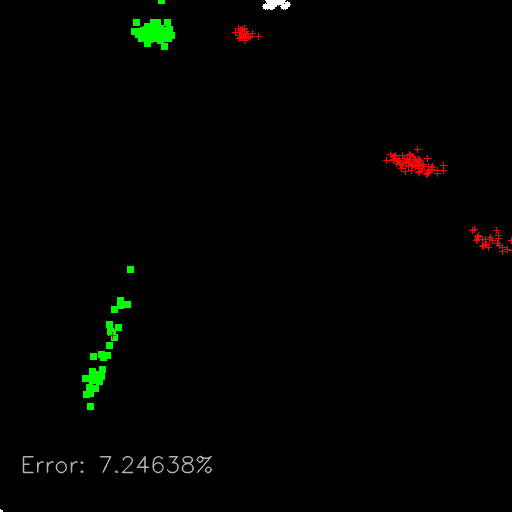# 输入图像预测1. 首先，我们加载图像并将其转换为灰色值。
2. 然后，我们使用preprocessImage函数应用预处理任务(如我们在第 5 章自动光学检查离子、对象分割和检测中了解到的)
    Mat pre= preprocessImage(img);

1. 现在，我们将使用前面描述的ExtractFeatures来提取图像中出现的所有对象的向量特征以及每个对象的左上角位置：
    // Extract features
vector<int> pos_top, pos_left;
vector< vector<float> >
features=ExtractFeatures(pre, &pos_left,     &pos_top);

1. 我们将检测到的每个对象存储为特征行，然后将每行转换为一行和两个特征的Mat
     for(int i=0; i< features.size(); i++){
Mat trainingDataMat(1, 2, CV_32FC1, &features[i]);

1. 之后，我们可以使用我们的StatModel支持向量机的predict函数对单个目标进行预测，预测的浮动结果就是检测到的目标的标签。 然后，要完成应用，我们必须在输出图像上绘制检测到并分类的每个对象的标签：
     float result= svm->predict(trainingDataMat);

1. 我们将使用stringstream存储文本，使用Scalar存储每个不同标签的颜色：
     stringstream ss;
Scalar color;
if(result==0){
color= green; // NUT
ss << "NUT";
}else if(result==1){
color= blue; // RING
ss << "RING" ;
}else if(result==2){
color= red; // SCREW
ss << "SCREW";
}

1. 我们还将使用在ExtractFeatures函数中检测到的位置在每个对象上绘制标签文本：
     putText(img_output,
ss.str(),
Point2d(pos_left[i], pos_top[i]),
FONT_HERSHEY_SIMPLEX,
0.4,
color);

1. 最后，我们将在输出窗口中绘制结果：
       miw->addImage("Binary image", pre);
miw->render();
waitKey(0);